# Perpendicular bisector and angle bisector Warm Up Construct

• Slides: 33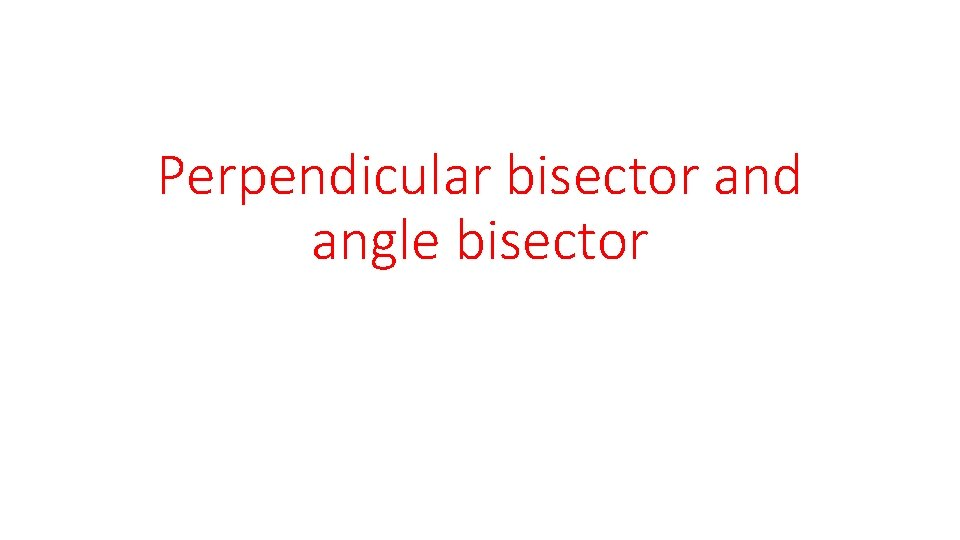Perpendicular bisector and angle bisector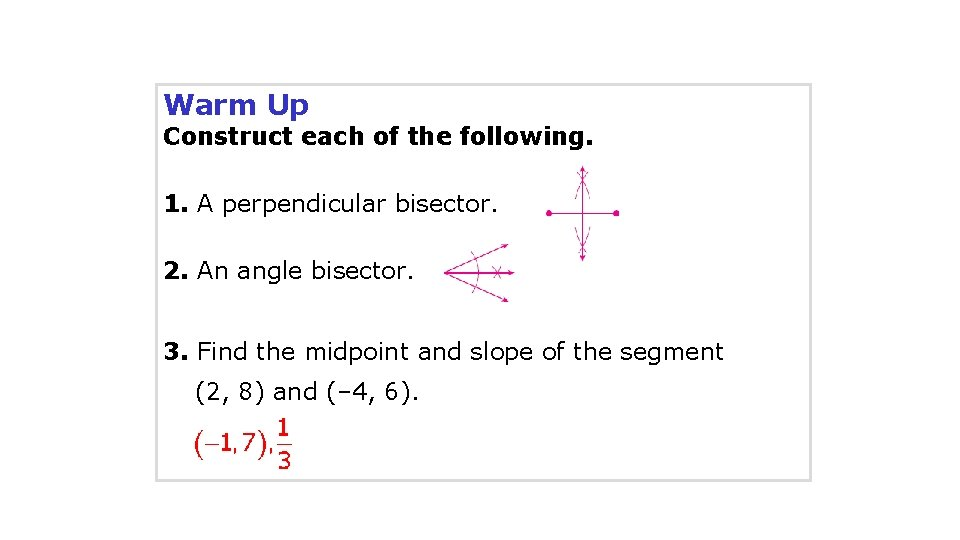Warm Up Construct each of the following. 1. A perpendicular bisector. 2. An angle bisector. 3. Find the midpoint and slope of the segment (2, 8) and (– 4, 6).Objectives Prove and apply theorems about perpendicular bisectors. Prove and apply theorems about angle bisectors.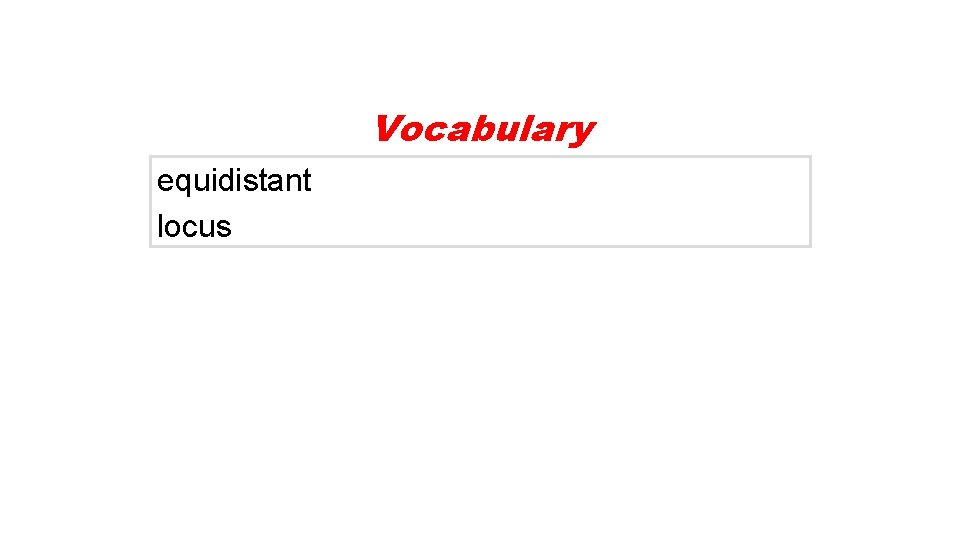Vocabulary equidistant locusWhen a point is the same distance from two or more objects, the point is said to be equidistant from the objects. Triangle congruence theorems can be used to prove theorems about equidistant points.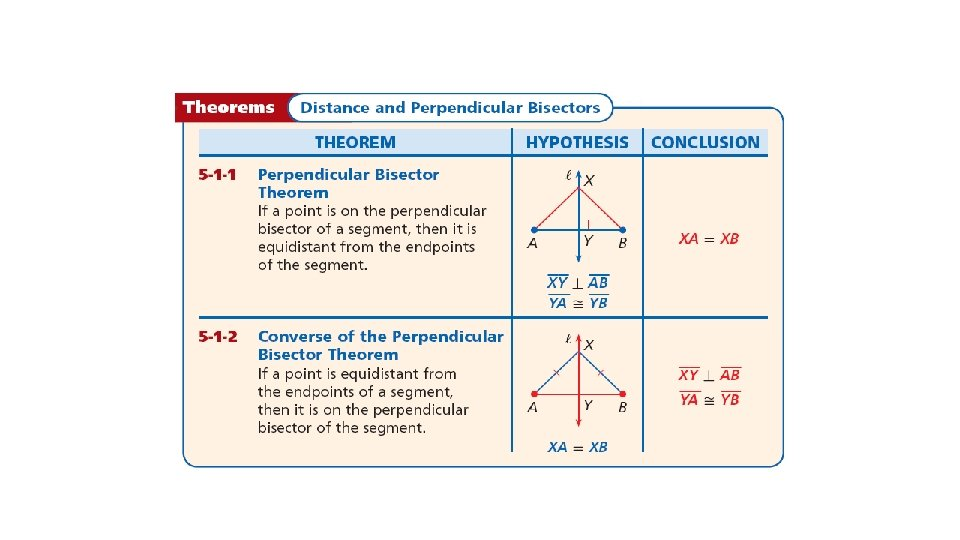A locus is a set of points that satisfies a given condition. The perpendicular bisector of a segment can be defined as the locus of points in a plane that are equidistant from the endpoints of the segment.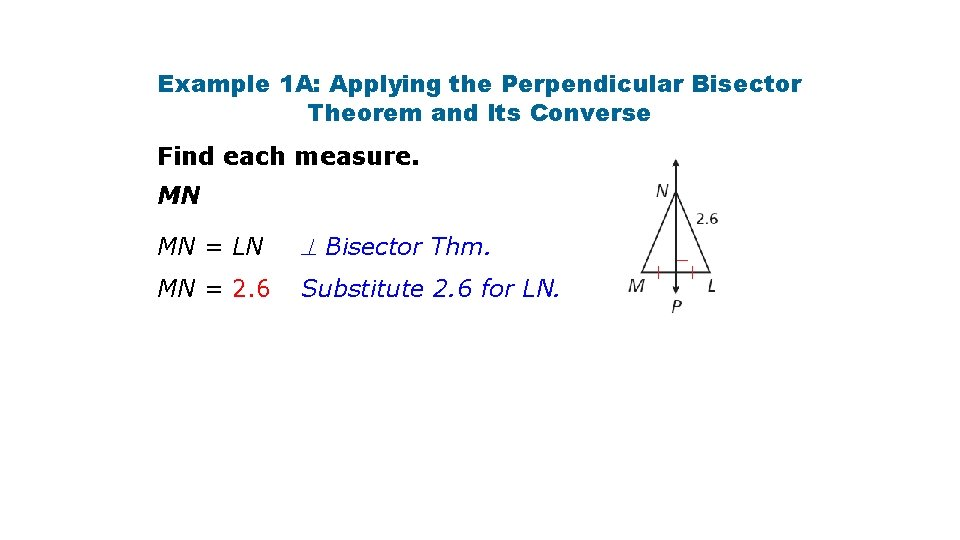Example 1 A: Applying the Perpendicular Bisector Theorem and Its Converse Find each measure. MN MN = LN Bisector Thm. MN = 2. 6 Substitute 2. 6 for LN.Example 1 B: Applying the Perpendicular Bisector Theorem and Its Converse Find each measure. BC Since AB = AC and , is the perpendicular bisector of by the Converse of the Perpendicular Bisector Theorem. BC = 2 CD Def. of seg. bisector. BC = 2(12) = 24 Substitute 12 for CD.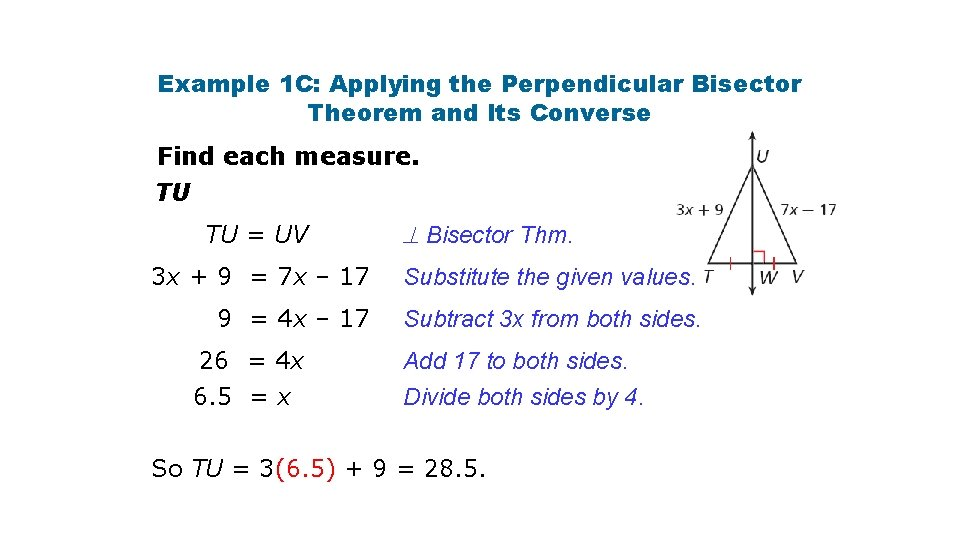Example 1 C: Applying the Perpendicular Bisector Theorem and Its Converse Find each measure. TU TU = UV Bisector Thm. 3 x + 9 = 7 x – 17 Substitute the given values. 9 = 4 x – 17 Subtract 3 x from both sides. 26 = 4 x 6. 5 = x Add 17 to both sides. Divide both sides by 4. So TU = 3(6. 5) + 9 = 28. 5.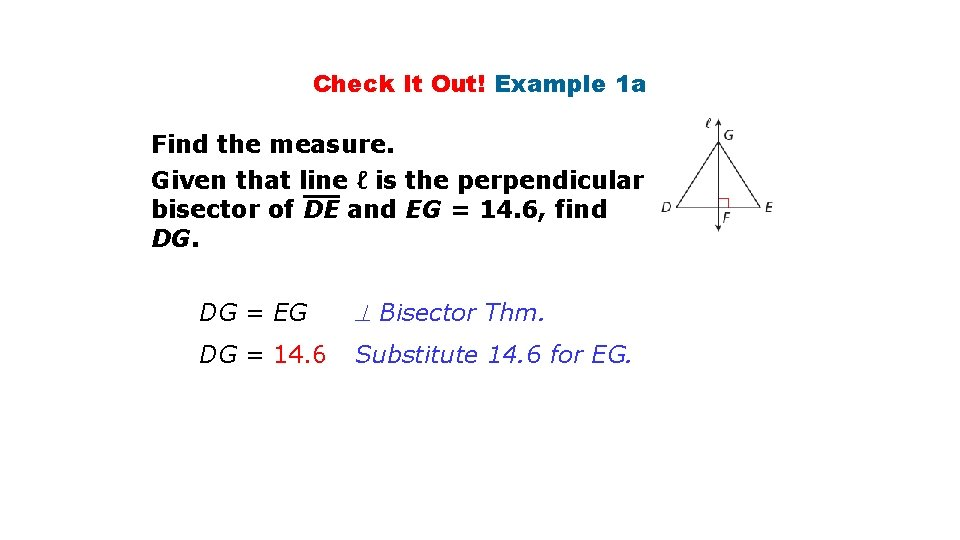Check It Out! Example 1 a Find the measure. Given that line ℓ is the perpendicular bisector of DE and EG = 14. 6, find DG. DG = EG Bisector Thm. DG = 14. 6 Substitute 14. 6 for EG.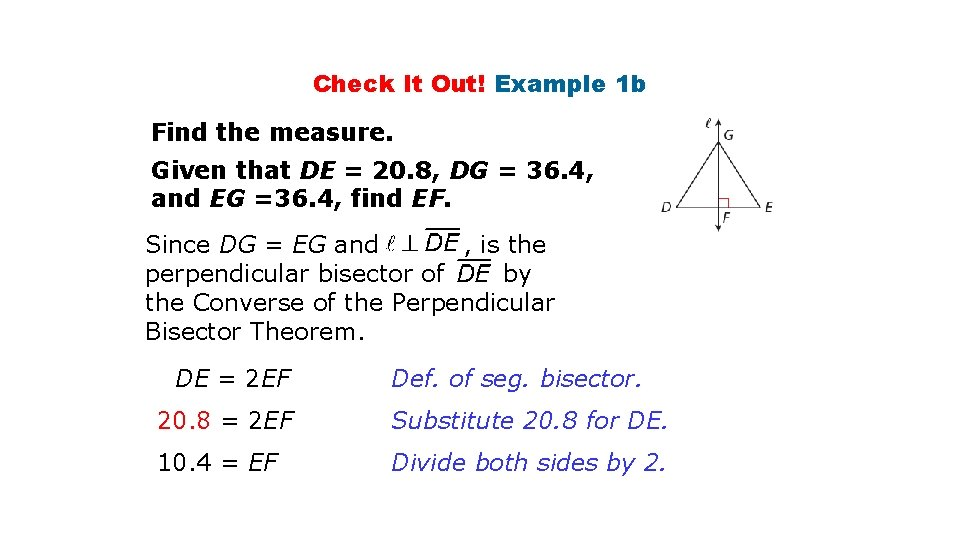Check It Out! Example 1 b Find the measure. Given that DE = 20. 8, DG = 36. 4, and EG =36. 4, find EF. Since DG = EG and , is the perpendicular bisector of by the Converse of the Perpendicular Bisector Theorem. DE = 2 EF Def. of seg. bisector. 20. 8 = 2 EF Substitute 20. 8 for DE. 10. 4 = EF Divide both sides by 2.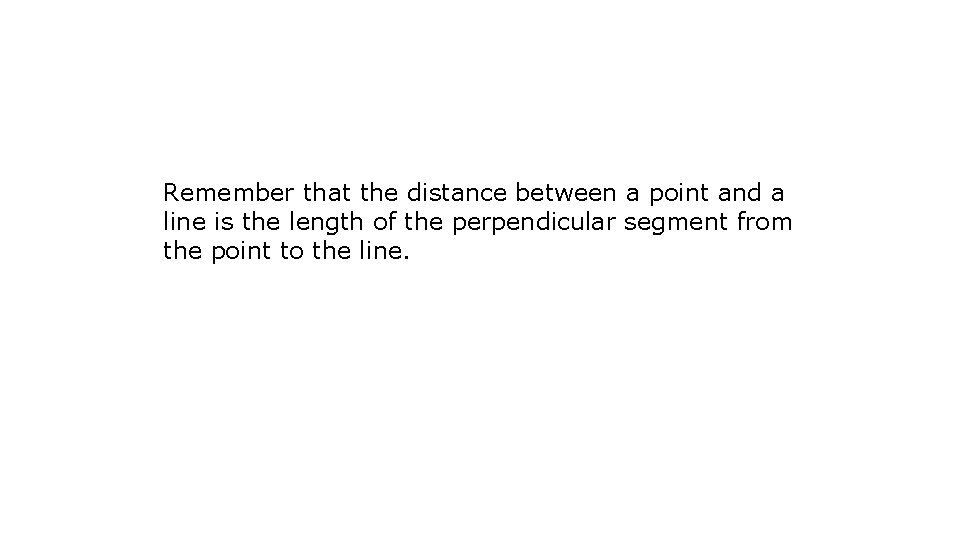Remember that the distance between a point and a line is the length of the perpendicular segment from the point to the line.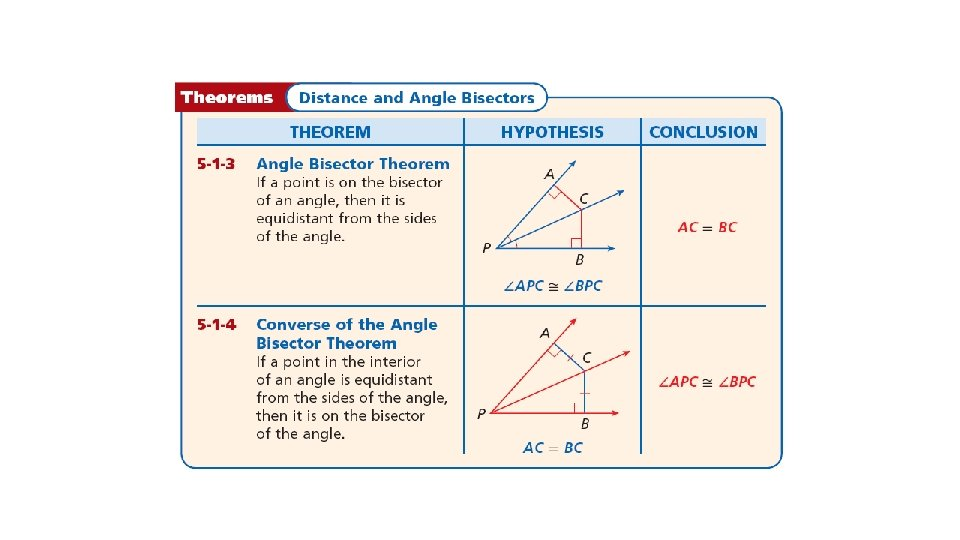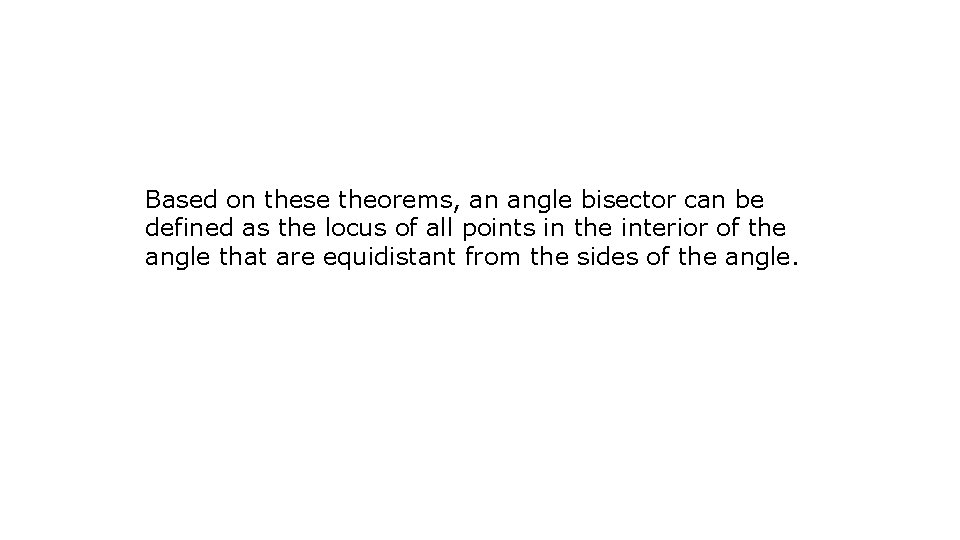Based on these theorems, an angle bisector can be defined as the locus of all points in the interior of the angle that are equidistant from the sides of the angle.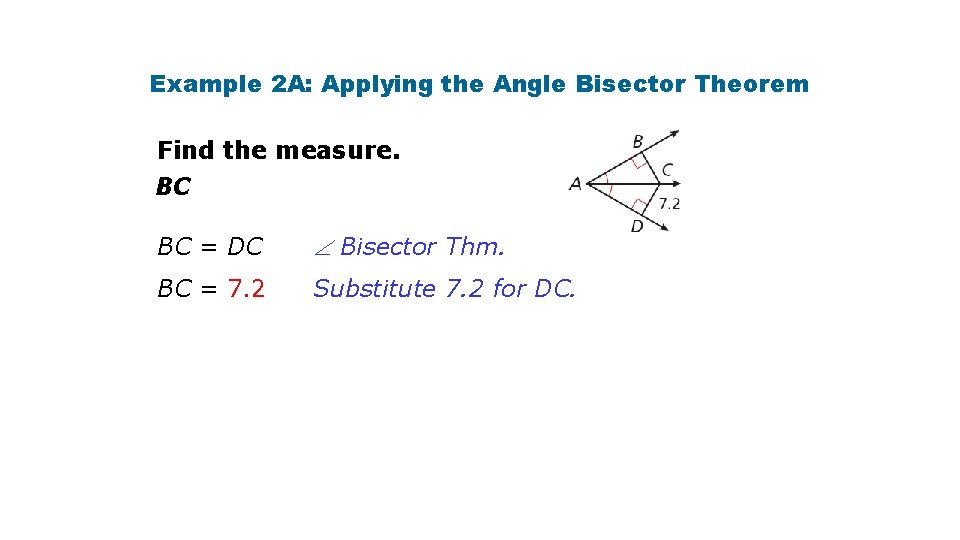Example 2 A: Applying the Angle Bisector Theorem Find the measure. BC BC = DC Bisector Thm. BC = 7. 2 Substitute 7. 2 for DC.Example 2 B: Applying the Angle Bisector Theorem Find the measure. m EFH, given that m EFG = 50°. Since EH = GH, and , bisects EFG by the Converse of the Angle Bisector Theorem. Def. of bisector Substitute 50° for m EFG.Example 2 C: Applying the Angle Bisector Theorem Find m MKL. Since, JM = LM, and , bisects JKL by the Converse of the Angle Bisector Theorem. m MKL = m JKM Def. of bisector 3 a + 20 = 2 a + 26 a + 20 = 26 a=6 Substitute the given values. Subtract 2 a from both sides. Subtract 20 from both sides. So m MKL = [2(6) + 26]° = 38°Check It Out! Example 2 a Given that YW bisects XYZ and WZ = 3. 05, find WX. WX = WZ Bisector Thm. WX = 3. 05 Substitute 3. 05 for WZ. So WX = 3. 05Check It Out! Example 2 b Given that m WYZ = 63°, XW = 5. 7, and ZW = 5. 7, find m XYZ. m WYZ + m WYX = m XYZ m WYZ = m WYX m WYZ + m WYZ = m XYZ 2(63°) = m XYZ 126° = m XYZ Bisector Thm. Substitute m WYZ for m WYX. Simplify. Substitute 63° for m WYZ. Simplfiy.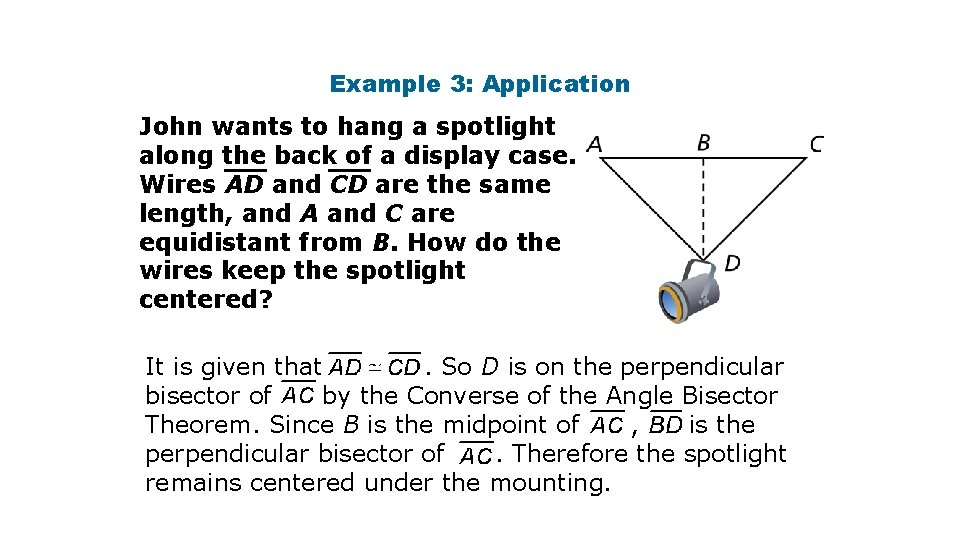Example 3: Application John wants to hang a spotlight along the back of a display case. Wires AD and CD are the same length, and A and C are equidistant from B. How do the wires keep the spotlight centered? It is given that. So D is on the perpendicular bisector of by the Converse of the Angle Bisector Theorem. Since B is the midpoint of , is the perpendicular bisector of. Therefore the spotlight remains centered under the mounting.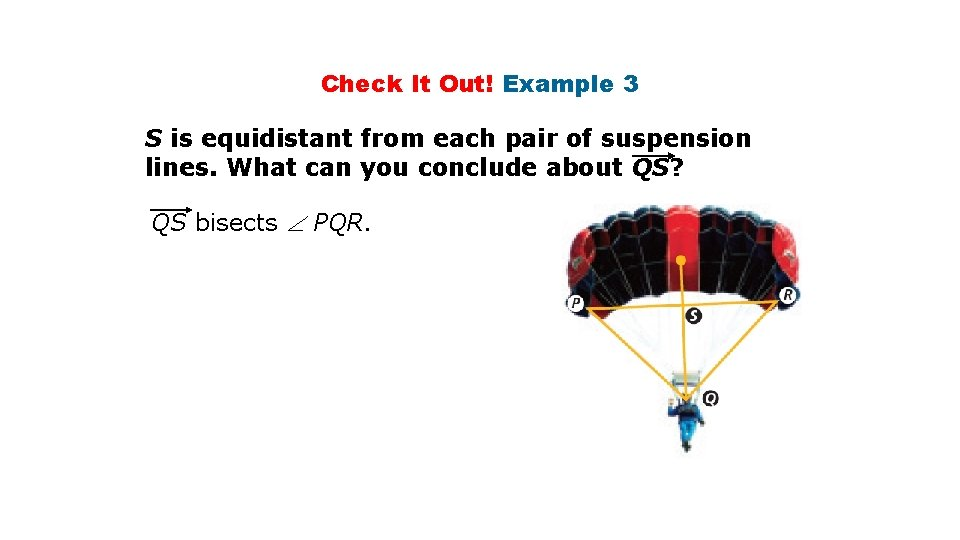Check It Out! Example 3 S is equidistant from each pair of suspension lines. What can you conclude about QS? QS bisects PQR.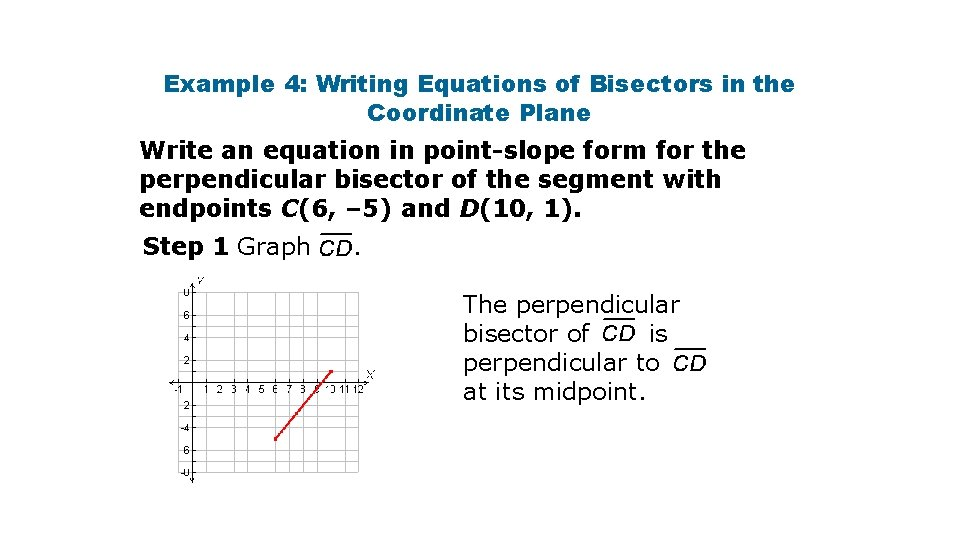Example 4: Writing Equations of Bisectors in the Coordinate Plane Write an equation in point-slope form for the perpendicular bisector of the segment with endpoints C(6, – 5) and D(10, 1). Step 1 Graph . The perpendicular bisector of is perpendicular to at its midpoint.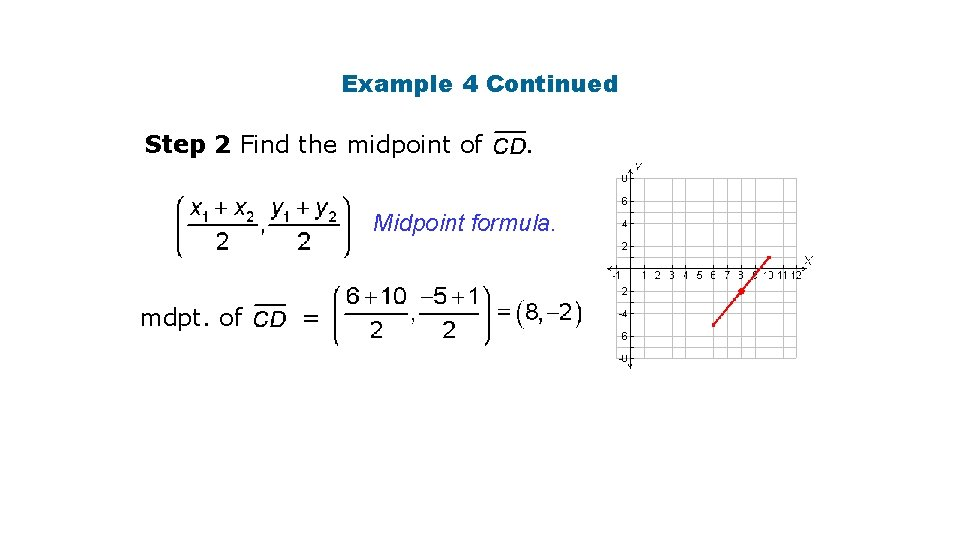Example 4 Continued Step 2 Find the midpoint of . Midpoint formula. mdpt. of =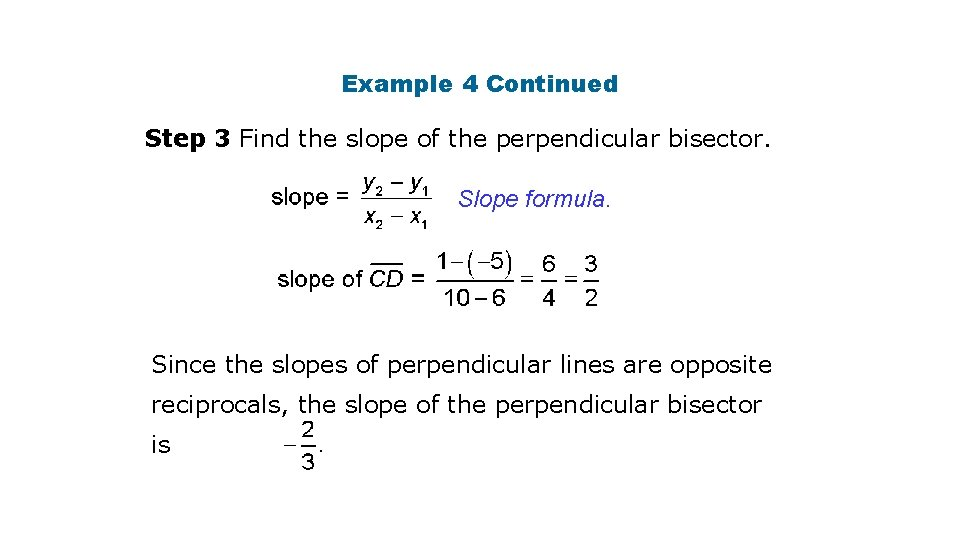Example 4 Continued Step 3 Find the slope of the perpendicular bisector. Slope formula. Since the slopes of perpendicular lines are opposite reciprocals, the slope of the perpendicular bisector isExample 4 Continued Step 4 Use point-slope form to write an equation. The perpendicular bisector of has slope and passes through (8, – 2). y – y 1 = m(x – x 1) Point-slope form Substitute – 2 for y 1, for x 1. for m, and 8Example 4 ContinuedCheck It Out! Example 4 Write an equation in point-slope form for the perpendicular bisector of the segment with endpoints P(5, 2) and Q(1, – 4). Step 1 Graph PQ. The perpendicular bisector of is perpendicular to at its midpoint.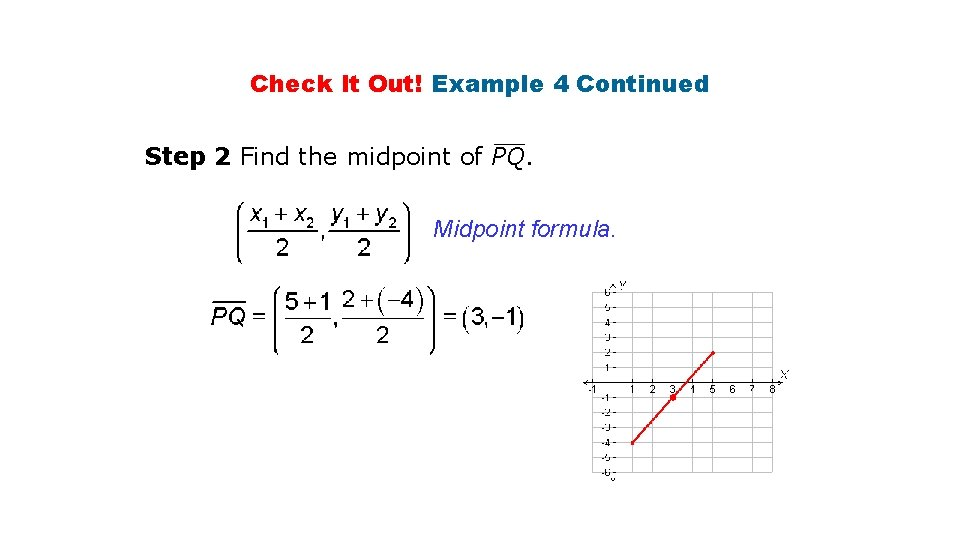Check It Out! Example 4 Continued Step 2 Find the midpoint of PQ. Midpoint formula.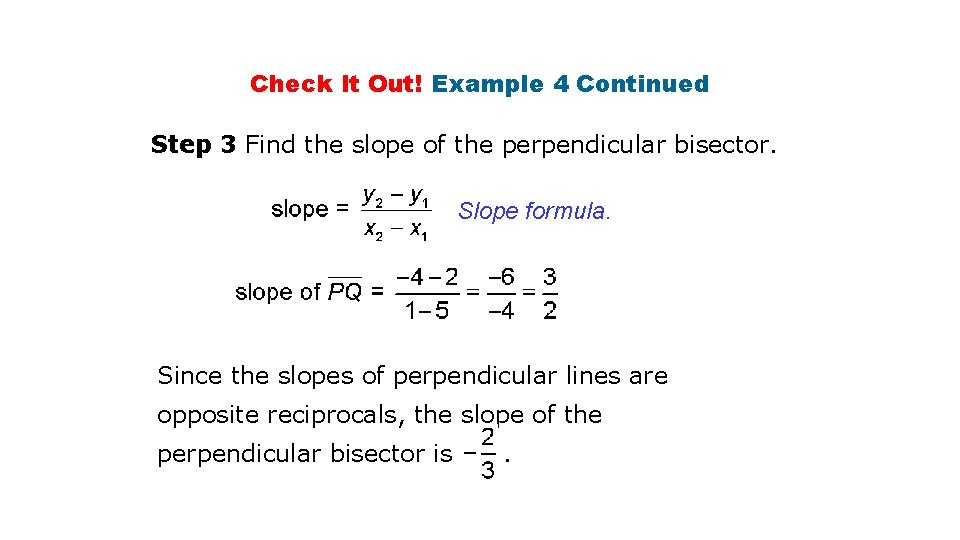Check It Out! Example 4 Continued Step 3 Find the slope of the perpendicular bisector. Slope formula. Since the slopes of perpendicular lines are opposite reciprocals, the slope of the perpendicular bisector is .Check It Out! Example 4 Continued Step 4 Use point-slope form to write an equation. The perpendicular bisector of PQ has slope passes through (3, – 1). y – y 1 = m(x – x 1) Point-slope form Substitute. andLesson Quiz: Part I Use the diagram for Items 1– 2. 1. Given that m ABD = 16°, find m ABC. 32° 2. Given that m ABD = (2 x + 12)° and m CBD = (6 x – 18)°, find m ABC. 54° Use the diagram for Items 3– 4. 3. Given that FH is the perpendicular bisector of EG, EF = 4 y – 3, and FG = 6 y – 37, find FG. 65 4. Given that EF = 10. 6, EH = 4. 3, and FG = 10. 6, find EG. 8. 6Lesson Quiz: Part II 5. Write an equation in point-slope form for the perpendicular bisector of the segment with endpoints X(7, 9) and Y(– 3, 5).